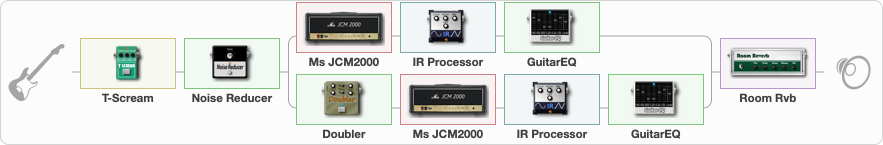# RideTheLigtning

Discussion in 'ToneLib-GFX presets' started by Serpov, Oct 16, 2022.

1. RideTheLigtning

Preset name: RideTheLigtning

Effects chain:Effect: "T-Scream" (Overdrive / Distortion), active - "yes"
{
"Drive" = 0
"Tone" = 100
"Level" = 100
}

Effect: "Noise Reducer" (Dynamics / Filter), active - "yes"
{
"Sens" = 66
"Mode" = Hard
}

Effect: "Splitter" (Dynamics / Filter)
{
"A-Bypass" = Off
"A-Pan" = -65
"A-Level" = 55
"B-Bypass" = Off
"B-Pan" = 65
"B-Level" = 55
"Width" = 100

'A' branch:
{

Effect: "Ms JCM2000" (Amp simulators), active - "yes"
{
"Gain" = 45
"Bass" = 45
"Middle" = 100
"Treble" = 60
"Presence" = 45
"Master" = 50
"Level (dB)" = 0
}

Effect: "IR Processor" (Cabinets), active - "yes"
{
"IR" = ride_L_IR
"Low Cut (Hz)" = 82
"Hi Cut (kHz)" = 10.0
"Mix" = 100
"Level (dB)" = 0
}

Effect: "GuitarEQ" (Dynamics / Filter), active - "yes"
{
"160 Hz" = 3
"400 Hz" = 0
"800 Hz" = -9
"1.6 kHz" = 0
"3.2 kHz" = 3
"6.4 kHz" = 1
"12 kHz" = 0
"Level (dB)" = 0
}
}
'B' branch:
{

Effect: "Doubler" (Dynamics / Filter), active - "yes"
{
"A-Dly" = 70
"A-Inv" = On
"A-Level" = 80
"B-Dly" = 50
"B-Inv" = On
"B-Level" = 80
}

Effect: "Ms JCM2000" (Amp simulators), active - "yes"
{
"Gain" = 45
"Bass" = 45
"Middle" = 100
"Treble" = 60
"Presence" = 45
"Master" = 50
"Level (dB)" = 0
}

Effect: "IR Processor" (Cabinets), active - "yes"
{
"IR" = ride_R_IR
"Low Cut (Hz)" = 82
"Hi Cut (kHz)" = 10.0
"Mix" = 100
"Level (dB)" = 0
}

Effect: "GuitarEQ" (Dynamics / Filter), active - "yes"
{
"160 Hz" = 3
"400 Hz" = 0
"800 Hz" = -9
"1.6 kHz" = 0
"3.2 kHz" = 3
"6.4 kHz" = 1
"12 kHz" = 0
"Level (dB)" = 0
}
}
}

Effect: "Room Rvb" (Reverberation), active - "yes"
{
"Time" = 4.5
"PreDelay" = 0
"LoDamp" = 46
"HiDamp" = 25
"Mix" = 30
}

Note: You will need to download and install the ToneLib-GFX software to use the preset.

File size:
3.6 KB
Views:
2,712